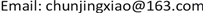LDA，协同过滤，主题模型，推荐系统, LDA Collaborative Filtering Topic Model Recommendation System1. 引言

2. 基于主题模型的用户兴趣变化模型的建立

2.1. 基于用户历史划分的新闻主题分布

LDA (Latent Dirichlet Allocation)是用于挖掘文本隐结构的重要的概率主题模型。本文利用LDA挖掘用户新闻阅读历史的隐结构作为用户兴趣  。

P ( I u i , j t l ) = ∑ k = 1 K P ( I u i , j t l | T i = k ) P ( T i = k ) (1)

Φ i j t l = P ( I i | T j , t l ) = C i j N K + β ∑ i = 1 N C i j N K + N β (2)

θ i j t l = P ( T j | u i , t l ) = C i j M K + α ∑ k = 1 K C i k M K + K α (3)

P u i = { P u i t 1 , P u i t 2 , ⋯ , P u i t | t u i | } 。

2.2. 基于时间惩罚权值的用户阅读兴趣变化模型

f u i ( t n ) = e − 1 | t u i | ( | t u i | − n ) (4)

P u i t | t u i | + 1 = f u i ( t 1 ) P u i t 1 + f u i ( t 2 ) P u i t 2 + ⋯ + f u i ( t | t u i | ) P u i t | t u i | (5)

2.3. 热点新闻/话题推荐

s i m ( P u i , P n j ) = cos ( P u i , P n j ) = P u i ⋅ P n j | P u i | | P n j | (6)

3. 实验与结果分析3.1. 数据集

3.2. 评估标准

Precision = ∑ u | R ( u ) ∩ T ( u ) | ∑ u | R ( u ) | (7)

Recall = ∑ u | R ( u ) ∩ T ( u ) | ∑ u | T ( u ) | (8)

3.3. 参数敏感性分析

3.4. 性能评估

Comparing the accuracy rate of number of different topics between LDA and LDA-C

LDA0.220.280.320.240.320.31
LDA-CF0.260.310.370.280.340.33

3.5. 推荐案例

4. 总结